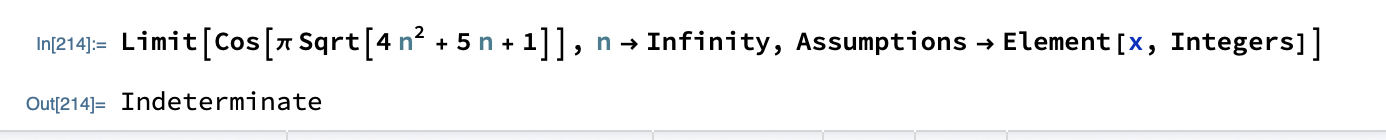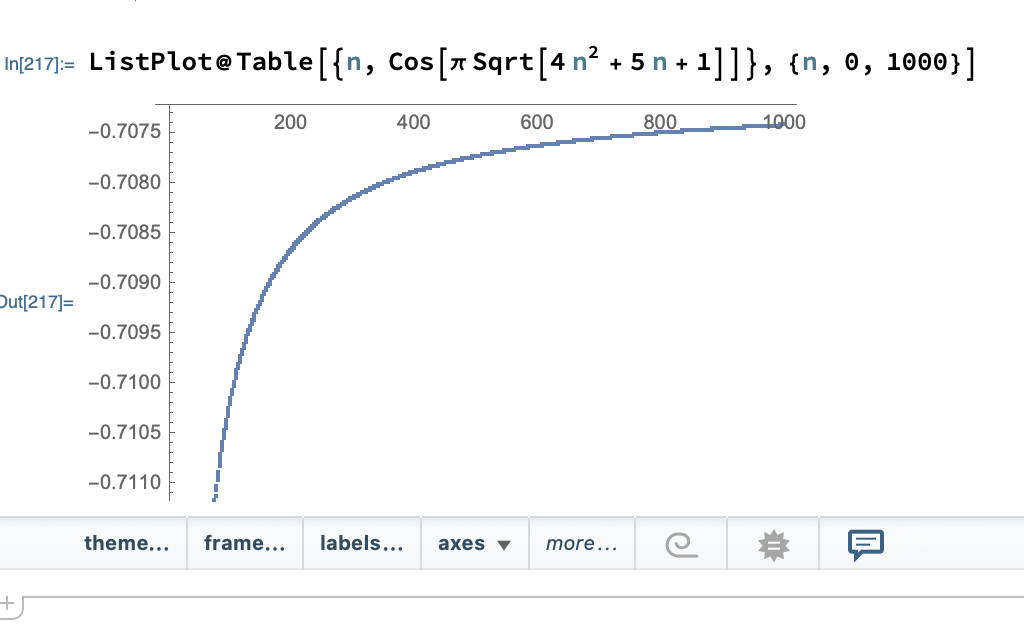# Computing the limit $\lim_{n\to\infty} \cos\left( \pi \sqrt{4n^2 + 5n + 1} \right)$ for $n \in \Bbb z$

I want to compute the limit

$$\qquad \lim \limits_{n\to\infty} \cos\left( \pi \sqrt{4n^2 + 5n + 1} \right)$$

for integer $$n$$. By completing the square, we can determine that this limit is equal to $$- \tfrac1{\sqrt2} \approx -0.7071$$.

But if we don't restrict $$n$$ to an integer, then the limit is indeterminate / does not exist. And can be easily found by typing it on WolframAlpha. Or in Mathematica:However, I do not know how to compute the limit (on Mathematica) with the original constraint that $$n$$ must be an integer.

I know that we can plot a graph on Mathematica:The graph suggests that the limit is equal to $$-\tfrac1{\sqrt2}$$. However, this doesn't look like a convincing result because we can't know that the limit is exactly equal to $$-\tfrac1{\sqrt2}$$.

Question: Is there a way to compute this limit in Mathematica where it spits out a single numerical value (of $$-1/{\sqrt2}$$)?

• Actually, can you show the analytical derivation of the result? It should help also coming up with how to coerce Mathematica into solving the limit.
– Kiro
Oct 9, 2020 at 10:11
• Hi Kiro. Karen has posted an analytical solution here. If you're too lazy to sign in to see the solution, just see this image. Oct 9, 2020 at 11:19
• Thank you (user64494, Vaclav, and Kiro) for your swift response. This is the first time I'm posting on Mathematica Stackexchange, and I'm happy to see such an active and helpful community! Oct 9, 2020 at 11:28
• I will just point out to you that you are not allowed to do any Assumptions in Limit that involves n (you did also chose x by mistake), only other variables (and it does warn about it). But the older version of Limit does allow that. So you can just do it: AsymptoticsClassicLimit[Cos[Pi*Sqrt[4 n^2 + 5 n + 1]], n -> \[Infinity], Assumptions -> n \[Element] Integers] it does not work in more complex cases though, like in mathematica.stackexchange.com/questions/43932/limit-of-sequence Jun 2, 2022 at 10:33
• In 13.2 DiscreteLimit[Cos[Pi*Sqrt[4 n^2 + 5 n + 1]], n -> \[Infinity]] works! Dec 27, 2022 at 14:14

DiscreteLimit[Normal[Series[Cos[Pi*Sqrt[4 n^2 + 5 n + 1]],{n,Infinity,1}]],n -> Infinity]
(*-(1/Sqrt)*)

• This is what I'm looking for. In fact, I was hoping to generalize my question where the domain of $n$ is of my own choosing. For example, if $n$ is half or any integer, or if $n$ can only be a prime number, then the limit remains the same. Let me try to tweak your code to see how to get this done. On the other hand, feel free to leave some hints here to steer me in the right direction. Oct 9, 2020 at 11:25
• Bug in DiscreteLimit! Wow!! It does not work when it is bare, which is very strange, since AsymptoticsClassicLimit[Cos[Pi*Sqrt[4 n^2 + 5 n + 1]], n -> \[Infinity], Assumptions -> n \[Element] Integers] does work. Jun 2, 2022 at 10:35
• In 13.2 DiscreteLimit[Cos[Pi*Sqrt[4 n^2 + 5 n + 1]], n -> \[Infinity]] works! Dec 27, 2022 at 14:10
DiscreteAsymptotic[Cos[π*
Sqrt[4*n^2 + 5 n + 1]], n -> Infinity](*-(1/Sqrt)*)

• Thank you too, A little mouse on the pampas. Oct 9, 2020 at 11:46
• +1.Moreover, DiscreteAsymptotic[Cos[\[Pi]*Sqrt[4*n^2 + 5 n + 1]], n -> Infinity, SeriesTermGoal -> 2] produces three terms $$\frac{\frac{81 \pi ^2}{8192 \sqrt{2}}+\frac{45 \pi }{512 \sqrt{2}}}{n^2}-\frac{9 \pi }{64 \sqrt{2} n}-\frac{1}{\sqrt{2}} ,$$ not two ones (despite the documentation) as a bonus. Oct 9, 2020 at 15:27
• "despite the documentation" But you can put constant in either of the other terms. Dec 27, 2022 at 14:22

We have

 Normal[Series[Sqrt[4*n^2 + 5 n + 1], {n, Infinity, 1}]]
(* 5/4 - 9/(64 n) + 2 n *)


If n tends to infinity, the expression above tends to

 Cos[(5/4 + 2 n) \[Pi]]


For integer n is the limit equal to

$$\cos \left(\frac{5 \pi }{4}\right)=-\frac{1}{\sqrt{2}}$$

• Thank you for your solution. I'm reluctant to edit the expression of the limit because I wanted to have the ability to know how to directly compute limits like this (for integer $n$) on Mathematica without manipulating the expression. Oct 9, 2020 at 11:21

A bit of a patchwork way to show that the limit is indeed -1/Sqrt for integer values of n goes as follows.

We make a series expansion of Sqrt[4n^2+5n+1] at infinity.

ser = Series[Sqrt[4 n^2 + 5 n + 1], {n, \[Infinity], 3}];


Then by keeping in mind that Cos is periodic with a period of 2 Pi, we can do

Cos[Limit[ser - 2 n, n -> \[Infinity]] Pi]
(*-(1/Sqrt)*)


where ser-2n is effectively taking the result modulo 2Pi and is valid if and only if n is an integer.

I admit that this is a bit sketchy and unsatisfactory way of doing this. While we can fully justify what we do, if we made a mistake in our thinking then the result is also wrong, so correctness of the result depends significantly on things not taken care of by Mathematica. I expect there to be more elegant ways.

• I did not consider converting the expression into a series in Mathematica at all. Thank you for showing me an alternative approach. Oct 9, 2020 at 11:27